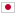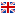Contest Duration: - (local time) (180 minutes) Back to Home
D - Maze/Time Limit: 2 sec / Memory Limit: 256 MB

### Problem

There is a maze which has 1 start and 2 goals. The maze is made of H rows and W columns rectangular array of cells. Each cell is specified as start, goal, pathway, or an obstacle. Let (r, c) be the r-th row's, c-th cell from the left.

You will be given a map of the maze. You have to draw two paths from start cell to each goal cell in that map. A path is an array of cells that begins with start cell and ends with goal cell, contains no obstacle cell, and each pair of neighboring cells in the array is adjoining. A pair of cells (a, b) and (c, d) is adjoining if |a-c|+|b-d|=1. The paths you draw must fulfill the following conditions.

• Each path must contain the least possible number of cells. That means each path must be the shortest path from start to each goal.
• To make paths distinguishable, each path must not have any cell in common, except the start cell.

Determine if you can make the paths that meets the condition in the given maze, and show the paths in the maze if possible. Read the Input and Output section for the detailed format.

### Input

H W
s_{(1,1)}s_{(1,2)}…s_{(1,W)}
s_{(2,1)}s_{(2,2)}…s_{(2,W)}
:
s_{(H,1)}s_{(H,2)}…s_{(1,W)}

• On the first line, two integers H, W (1 \leq H, W \leq 50), the size of the maze is given.

• On the following H lines, each line containing a string of length W, the map of the maze is given. The i-th (1 \leq i \leq H) line's j-th (1 \leq j \leq W) character represents the cell (i, j). Each character means as following.

• . represents that the cell is a pathway cell.
• # represents that the cell is an obstacle cell.
• S represents that the cell is the start cell.
• A represents that the cell is the first goal cell.
• B represents that the cell is the second goal cell.

It is guaranteed that the character S, A, B appears only once. Also it is guaranteed that for each goal there's at least 1 path.

### Output

If there are no paths to fulfill the conditions in the problem section, output 1 line containing NA.

If there are paths that fulfill the conditions, Output H lines that each line consists of W characters. The i-th (1 \leq i \leq H) line's j-th (1 \leq j \leq W) character in the output should represent the cell (i, j) in the maze. The output must fulfill the following conditions.

• The cell that was a pathway in the input must be one of ., a or b.
• The cell that was a start, an obstacle, or a goal cell in the input must be the same character as in the input.
• It should be able to reach the goal cell A from the start cell by following the adjoining a cell. The number of cell a must be the least possible to reach the goal.
• It should be able to reach the goal cell B from the start cell by following the adjoining b cell. The number of cell b must be the least possible to reach the goal.

In other words, show the path to each goal A, B by the characters a, b. If there are more than one possible answers, you may choose any one of them.

Make sure to insert a line break at the end of the output.

### Input Example 1

5 5
..#..
.B.A#
...#.
.....
..S#.


### Output Example 1

..#..
.BaA#
.ba#.
.ba..
.bS#.


### Input Example 2

2 3
SBA
...


### Output Example 2

NA


The goal cell is not an obstacle, so the shortest path to the goal A is only (1, 1)(1, 2)(1, 3). However, that path contains the goal cell B and thus you cannot avoid to use the common cell in the shortest paths. Therefore, you should output NA.

### Input Example 3

5 5
..#..
.B.A#
.#.#.
.....
..S#.


### Output Example 3

NA


### Input Example 4

5 8
.#...#..
.#.#.#..
.S.#...B
.#####A.
........


### Output Example 4

.#bbb#..
.#b#b#..
aSb#bbbB
a#####A.
aaaaaaa.